# Distance-Measures¶

## chebyshev - (chebyshev)¶

The distance is calculated as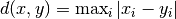.

## euclidean - (euclidean)¶

The distance is calculated as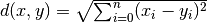.

## hamming - (hamming)¶

The distance is calculated as the number of components which differ over the number of components.

## manhattan - (manhattan)¶

The distance is calculated as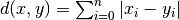.

## minkowski - (minkowski)¶

The distance is calculated as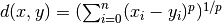.

• name: p
• min: 0.001
• default:
• type: float

## seuclidean - (seuclidean)¶

The distance is calculated as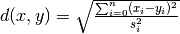, whereis the standard deviation between the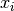andin the data set.

## sqeuclidean - (sqeuclidean)¶

The distance is calculated as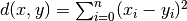.

## weuclidean - (weuclidean)¶

The distance is calculated as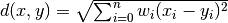.

### Parameters¶

#### weights - Comma-separated list of weights for each dimension.¶

• name: Weigths
• default:
• type: string

## wminkowski - (wminkowski)¶

The distance is calculated as.

### Parameters¶

• name: p
• min: 0.001
• default:
• type: float

#### weights - Comma-separated list of weights for each dimension.¶

• name: Weights
• default:
• type: string